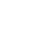# IC555 Calculator Online IC555 Astable Oscillator calculator

Hello Guys. I know that everyone wants to save time. I also know that sometimes time is limited and it’s a hassle to get to the calculation part before designing your IC555 based Oscillators, frequency, and pulse generator circuits. Or oscillators using IC555. Every time leaving work and putting time on calculations between work. So, here is a complete IC555 Calculator online tool. Using this online IC555 calculator tool you have to insert just some 3 values of R1, R2, and C. And, You’ll get the whole calculation outputs like the Frequency, Duty Cycle, T1, T0 and Time period. So, friends below is a tested IC555 calculator Online tool with by Electroinvention to help you guys save time and energy.

Also, with a standard IC555 Astable circuit configuration diagram. Followed by the whole explanation about formulas and terms like frequency, Duty cycle, Time high/Low, Time period, etc.

## Formulas, Terms & Explanations

Above is an IC555 Astable configured circuit with the Calculator tool. Hope you liked using this. Let me elaborate some Terms and formulas used in this Calculator tool below briefly.

Astable means it has no specifically stable states and the output is in a periodic alternating pulse that oscillates between the VCC and -ve(0). This means high-low periodically with a frequency.

The frequency can be adjusted by changing the values of R1, R2 and C.

1.Frequency:- It is the number of pulses per second. Or, the number of oscillations per second. The formula to calculate the frequency is given below

Frequency(Hz) =1.44 ⁄ ((R1+2R2)*C)

2.Time Period or Total Time period:- Time period is the total time covered for one pulse. Formula is given

T=1/F=0.694(R1+2R2)C

3.Time high and Time Low, i.e T1 & T2 are the Time high (ON) and Time Low (OFF).

T1=0.694(R1+R2)*C

T0=0.694*R2*C

The Time Period is the sum of Time High(T1) and Time Low(T0). Both are measured in milliseconds (ms).

4. Duty Cycle:- Duty cycle is the time ratio which is calculated in percent. This means how much time fraction the machine or signal is active and is in ON state. Suppose the Duty cycle is 95%, then 95% of the time the IC will give positive voltage. 95 % of the time span is ON state and voltage is +ve.

2nd example is if we have a 50% duty cycle( Although not possible to get exact 50% duty cycle), then 50% of the time span is High time, and the rest 50% will be the T0( Time Low). Formula to calculate duty Cycle is Below

D=Duty cycle= T1 /T× 100

5.Mark Space Ratio is the ratio of T1 and T0. It means ratio of positive part of amplitude to the negative.

Mark space ratio= T1 ⁄ T0

#### Some Important Tips and notes:-

• According to the 3 values inserted, R1, R2, and C respectively, the rest of the factors like frequency and duty cycle are set.
• Changing these values you can set accordingly and also can get to know what value of components are to be used.
• When R1 is increased, High Time(T1) is also increased without affecting Low Time(T0).
• When R2 is increased, High time(T1) is increased and T0 is decreased also decreasing the Duty Cycle.
• C (On-Off Capacitor) is increased to increase the Time Period.

Hope you guys Loved it. Tell me in comments. Suggestions. How did this helped you? Comment it.

## 2 Replies to “IC555 Calculator Online IC555 Astable Oscillator calculator”

1.anilswami says:

that’s good ideas i interested

1.Aabhishek says:

Thanks for the appreciation